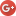# Investing for Beginners , investingAn advertising agency is 85 percent confusion and 15 percent commission.Fred Allen

## Investment Dictionary

Browse by search:

Browse by Letter: A B C D E F G H I J K L M N O P Q R S T U V W X Y Z All

## Internal Rate of Return

An internal rate of return (IRR) is a ratio used very often to measure a profitability of some investment project. IRR is determined as a discount rate when NPV of the project is equal to zero. If IRR is higher than cost of capital, then investment should be proceeded, but if cost of capital is higher than IRR of the project, then investment should be rejected, because it would destroy the value for shareholders, but not maximize it.

Internal rate of return is very popular among managers that are dilettantes in finance field because of this method simplicity. (The easiest way to calculate IRR is to use MS Excel’s IRR function).

Despite the simplicity of the IRR it should not be the only criteria for filtering investment projects. It would be advisable to calculate NPV, sensitivity analysis, riskiness and internal costs, alternative costs, future profitability ratios and dependability on KPIs, perform future cash flow analysis, analyze impact of changes in competition, fulfill market forecasts and other methods of financial analysis.

Despite the fact that IRR in many cases is over trusted, it is the one of most complete ratios compared to its simplicity. One of the disadvantages of this ratio is that internal rate of return do not adjusts to time effect to the value of money if an IRR isn’t positive (that may be important in more specific cases). However, IRR is a perfect ratio for fast calculation when numbers are clear. Another, yet simplified version of return rate is CAGR, which is calculated as geometric mean too.

See an example of internal rate of return calculation (using Excel formula IRR – input Net cash flow):

 Year 1 Year 2 Year 3 Year 4 IRR Investments 500 200 0 0 Return 0 100 300 500 Net cash flow -500 -100 300 500 12%

Read about other ratios or method’s that can be used in return analysis: